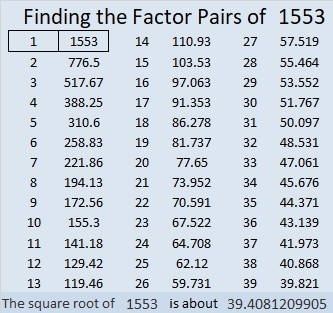# 1553 Ornamental Corn

Contents

### Today’s Puzzle:

Ornamental corn is a popular decoration at Thanksgiving. Today’s puzzle looks a little bit like ornamental corn, and there’s at least a kernel of truth to that statement! Solve the puzzle, and I will think YOU are a-maize-ing!Here’s the same puzzle if you want to print it in black and white:### Factors of 1553:

• 1553 is a prime number.
• Prime factorization: 1553 is prime.
• 1553 has no exponents greater than 1 in its prime factorization, so √1553 cannot be simplified.
• The exponent in the prime factorization is 1. Adding one to that exponent we get (1 + 1) = 2. Therefore 1553 has exactly 2 factors.
• The factors of 1553 are outlined with their factor pair partners in the graphic below.How do we know that 1553 is a prime number? If 1553 were not a prime number, then it would be divisible by at least one prime number less than or equal to √1553. Since 1553 cannot be divided evenly by 2, 3, 5, 7, 11, 13, 17, 19, 23, 29, 31, or 37, we know that 1553 is a prime number.

### More About the Number 1553:

1553 is the sum of the squares of two numbers that are reverses of each other:
32² + 23² = 1553

1553 is the hypotenuse of a primitive Pythagorean triple:
495-1472-1553, calculated from 32² – 23², 2(32)(23), 32² + 23².

Here’s another way we know that 1553 is a prime number: Since its last two digits divided by 4 leave a remainder of 1, and 32² + 23² = 1553 with 32 and 23 having no common prime factors, 1553 will be prime unless it is divisible by a prime number Pythagorean triple hypotenuse less than or equal to √1553. Since 1553 is not divisible by 5, 13, 17, 29, or 37, we know that 1553 is a prime number.

This site uses Akismet to reduce spam. Learn how your comment data is processed.